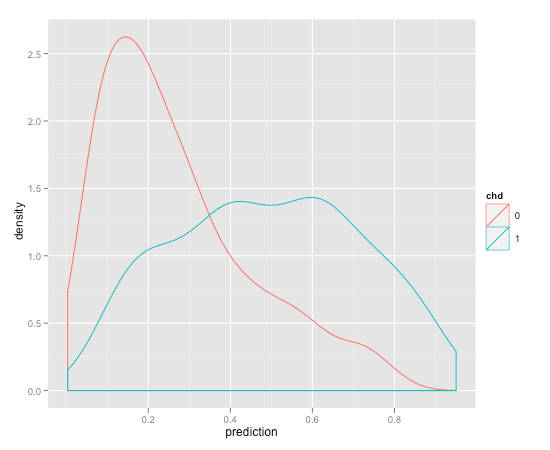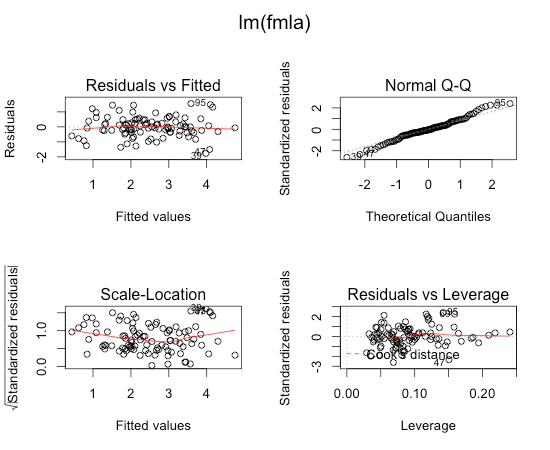# My Favorite Graphs

The important criterion for a graph is not simply how fast we can see a result; rather it is whether through the use of the graph we can see something that would have been harder to see otherwise or that could not have been seen at all.

— William Cleveland, The Elements of Graphing Data, Chapter 2

In this article, I will discuss some graphs that I find extremely useful in my day-to-day work as a data scientist. While all of them are helpful (to me) for statistical visualization during the analysis process, not all of them will necessarily be useful for presentation of final results, especially to non-technical audiences.

I tend to follow Cleveland’s philosophy, quoted above; these graphs show me — and hopefully you — aspects of data and models that I might not otherwise see. Some of them, however, are non-standard, and tend to require explanation. My purpose here is to share with our readers some ideas for graphical analysis that are either useful to you directly, or will give you some ideas of your own.

The graphs are all produced in `R`, using the `ggplot2` package. While `ggplot2` has a fairly high learning curve, it is the most flexible of the `R` graphing packages that I have encountered, and I’ve been able to quickly create rich graphics more easily than I would be able to with the `R` base graphics, or with other graphics packages.

Let’s start with some exploratory analysis. We will use the `AdultUCI` dataset that is included in the `arules` package.

``````
library(arules)
colnames(dframe) = c("education", "hours_per_week")
# get rid of the annoying minus signs in the column names
``````

We want to compare the distribution of work-week length to education, using a box-and-whisker plot that is overlaid on a jittered scatterplot of the data.

``````
library(ggplot2)
ggplot(dframe, aes(x=education, y=hours_per_week)) +
geom_point(colour="lightblue", alpha=0.1, position="jitter") +
geom_boxplot(outlier.size=0, alpha=0.2) + coord_flip()
``````

The `outlier.size=0` argument to `geom_boxplot` turns off the outlier plotting, and `coord_flip` switches the coordinate axes (because there are a lot of education levels).

The resulting graph:Recall that the box of a box-and-whisker plot covers the central 50% of the data distribution; the line in the center marks the median. In this case, the work-week length concentrates so strongly at 40 hours (except for PhDs and those with professional degrees; they are doomed to work longer hours, typically) that most of the boxes appear one-sided; it’s easier to see what is happening with both the scatterplot and box-and-whisker superimposed, than it might be with the box-and-whisker alone. We can also see the relative concentration of the subjects along each educational level.

I’ve found that this superimposed graph is fairly easy to explain in a presentation (easier than a plain box-and-whisker, actually). The primary disadvantage that the scatterplot can get illegible for high volume datasets (this set has about 49 thousand rows). In this case, we have to return to the box-and-whisker plot alone.

Beyond exploratory analysis, we also want plots to evaluate the models that we fit. Win-Vector’s bread-and-butter recently has been logistic regression, so we will start with some visualizations for evaluating binary logistic regression models. We’ll use the heart disease dataset that Hastie, et.al, used in the Elements of Statistical Learning.

``````
path = "http://www-stat.stanford.edu/~tibs/ElemStatLearn/datasets/SAheart.data"
fmla = "chd ~ sbp + tobacco + ldl + adiposity + famhist + typea + obesity"
na.action=na.exclude)
``````

We will make a data frame of chd (the true response, coronary heart disease), and the score from the model.

``````
dframe = data.frame(chd=as.factor(saheart\$chd),
prediction=predict(model, type="response"))
``````

The standard diagnostic plot for logistic models is the ROC curve, which is fine, but personally, I don’t get a visceral feel for the model from looking at the ROC. Also, if you are interested in setting a score threshold on the model for classification purposes, the ROC adds an additional level of indirection, since it essentially integrates the score away. I used to plot the distribution of score (prediction) versus true response, like so:

``````
ggplot(dframe, aes(x=prediction, colour=chd)) + geom_density()
``````This visualization tells me whether or not the model scores actually separate the response — in this case, the model identifies negative cases (no coronary heart disease) better than positive cases. The graph is hard to explain to a non-technical audience, and it has the disadvantage that both distributions are separately normalized to have unit area, so you get no sense of the relative proportion of positive and negative cases (in this case, about 35% of the population have coronary heart disease).

Here’s an alternate graph:

``````
ggplot(dframe, aes(x=prediction, fill=chd)) +
geom_histogram(position="identity", binwidth=0.05, alpha=0.5)
``````This is two semi-transparent histograms; the blue histogram for `chd=1` is “in front” of the the red histogram. Because they are histograms, rather than density plots, we can more clearly see the relative distribution of positive to negative cases, and we have a better sense of how well (or not) the model separates the positive cases from the negative ones. Clearly, for most score thresholds, the model will have a fairly high false positive rate. I use this visualization all the time, but it is also fairly hard to explain, the transparency in particular.

We can also use our friend the box-and-whisker scatterplot.

``````
ggplot(dframe, aes(x=chd, y=prediction)) +
geom_point(position="jitter", alpha=0.2) +
geom_boxplot(outlier.size=0, alpha=0.5)

``````The median score for the coronary heart disease cases is pulled away from the median score of the healthy subjects, but the central 50% of the two distributions still overlap.

Finally, let’s look at visualizations for linear regression. We’ll use the prostate cancer data from Elements of Statistical Learning.

``````
fmla = "lpsa ~ lcavol + lweight + age + lbph + svi + lcp + gleason + pgg45"
model = lm(fmla, data=prostate.data)
``````

We can just `plot(model)` for some diagnostic graphs:

``````
par(mfrow = c(2, 2), oma = c(0, 0, 2, 0))
plot(model)
``````
``` ```These diagnostics are useful to determine whether or not a linear model is suitable, and to identify outliers; but again, I personally don't get a visceral feel for the model. I prefer to directly plot prediction against true response:

``````
dframe = data.frame(lpsa=prostate.data\$lpsa, prediction=predict(model))

title = sprintf("Prostate Cancer modeln R-squared = %1.3f",
summary(model)\$r.squared)
ggplot(dframe, aes(x=lpsa, y=prediction)) +
geom_point(alpha=0.2) +
geom_line(aes(y=lpsa), colour="blue") +
opts(title=title)
``````
``` ```This graph gives you the same information as the Residuals vs. Fitted plot, and the Q-Q plot -- in particular, whether there is systematic over- or under-prediction in specific ranges of the data. It will expose outliers, and it is intuitive to explain when presenting your results. Furthermore, it can be used to evaluate other models that predict a continuous response, such as regression trees or polynomial fits.

Which graphs do you find especially useful for your day-to-day work?### Nina Zumel

Data scientist with Win Vector LLC. I also dance, read ghost stories and folklore, and sometimes blog about it all.

### 6 replies ›

1.Ian Fellows says:

I love to see the box and jitter plot getting some love. It is one of my GOTO plots. I also really like effect plots for logistic regression:

library(effects)
model.glm 0.0 ~ ldl*famhist,family=binomial(),data=saheart)
plot(effect(term=”ldl:famhist”,mod=model.glm,default.levels=20),rescale.axis=FALSE)

2.Ian Fellows says:

model.glm <- glm(formula=chd~ ldl*famhist,family=binomial(),data=saheart,na.action=na.omit)
plot(effect(term="ldl:famhist",mod=model.glm,default.levels=20),rescale.axis=FALSE)

3.Nina Zumel says:

Ah, very nice! Thanks, Ian.

Here’s the plot:

4.Naas says:

Thanks for the post. It’s very easy to understand and I’m sure that most predictive modeling practitioners will find it useful. I mostly use SAS but I’m very impressed by the flexibility and graphics capabilities of R.
They must just work on the processing power (utilizing more than one processor) and the connectivity to RDB’s. Both of which are good and easy in SAS but much more difficult in R

5.stefano ciotti says:

It’s a fantastic site. Well done!!!

6.jmount says:

Ran into a funny thing. I always liked the y as a function of prediction graph better than the traditional residual graph. This is despite Cleveland’s studies showing people are better at spotting structure versus a residual over spotting structure versus y=x. However I just ran into a teaching project where the residual graph really fails. If the residuals are normally distributed (no interesting structure) then the visual portion of the graph is completely scale invariant and fails to show scale. Without looking at the scale you can not tell if the residuals are large are small. This is in contrast to the y versus prediction graph where you can visually discern scale. I admit the scale should be there and should be read, but the visual aspect is failing if the information is literally not there until you read the scale.